• matlab画二维正态函数图像 画坐标网格 [x,y]=meshgrid(-5:0.1:5,-5:0.1:5) 正态分布密度函数 f=1/(2*pi*sigma1*sigma2*sqrt(1-p*p))*exp(-1/(2*(1-p*p))*(((x-u1).^2)./(sigma1*sigma1)-2*p*((x-u1)*(y-u2))./...
• 完全自己打码，实现了基于Matlab 实现二维正态分布贝叶斯决策面可视化，包括线性与非线性决策面； 种情况：①∑1 = ∑2 = σ^2*I，②∑1 = ∑2 ≠ σ^2*I，③∑1 ≠ ∑2。
• ## Matlab二维正态分布可视化

千次阅读 多人点赞 2019-04-22 13:11:44
高维分布一直都是一个很抽象的概念，本人在学的时候很多概念不太能理解，于是使用了Matlab进行了... 图1 三维图1 图2 俯视图1  变量x的均值为0，方差为2，变量y的均值为0，方差为2，相关系数为0。 ...
 高维分布一直都是一个很抽象的概念，本人在学的时候很多概念不太能理解，于是使用了Matlab进行了可视化。并对均值、方差以及相关系数进行了调整。  图中x,y为两个变量的取值，z为出现的概率密度。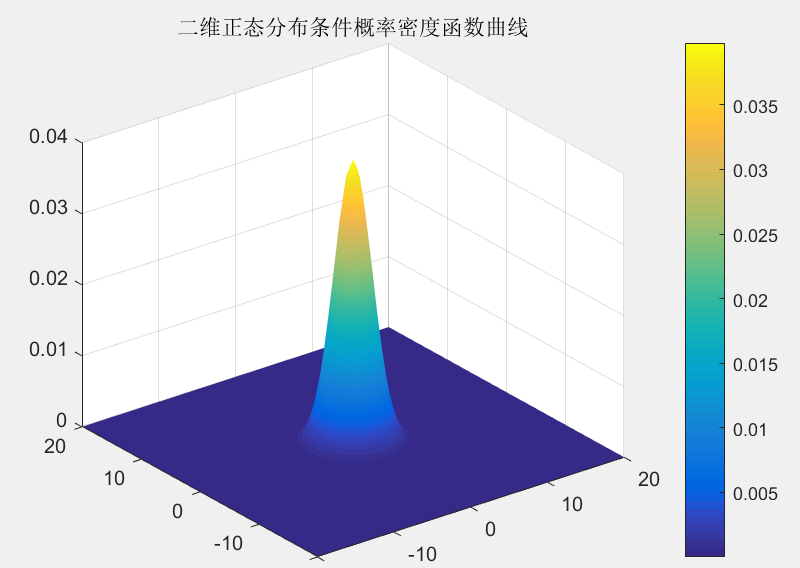图1 三维图1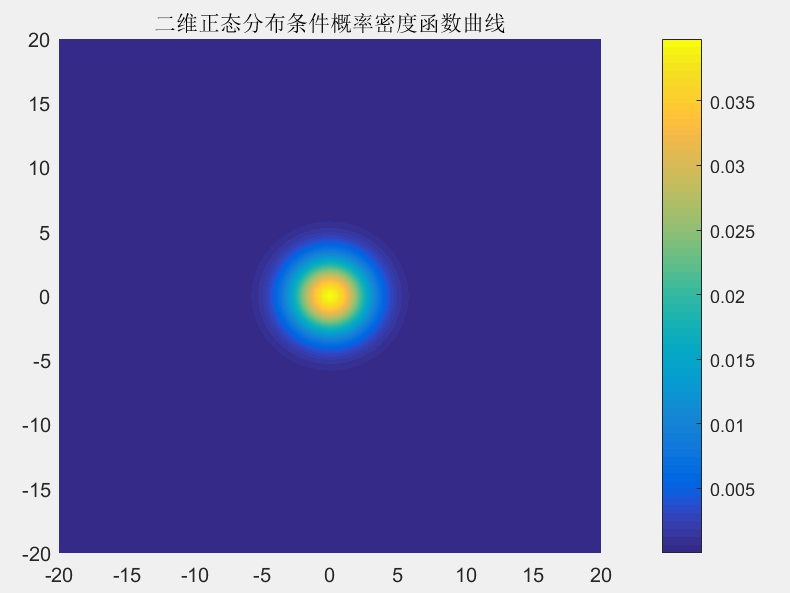图2 俯视图1
变量x的均值为0，方差为2，变量y的均值为0，方差为2，相关系数为0。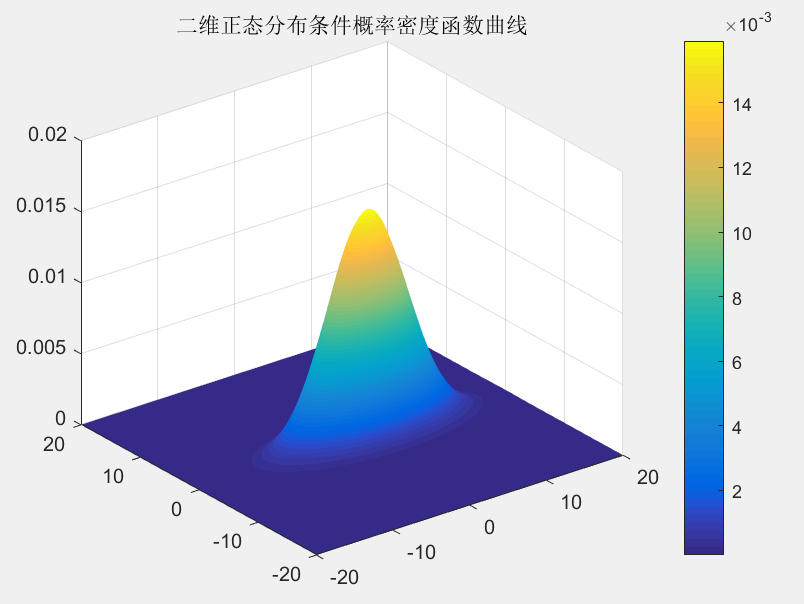图3 三维图2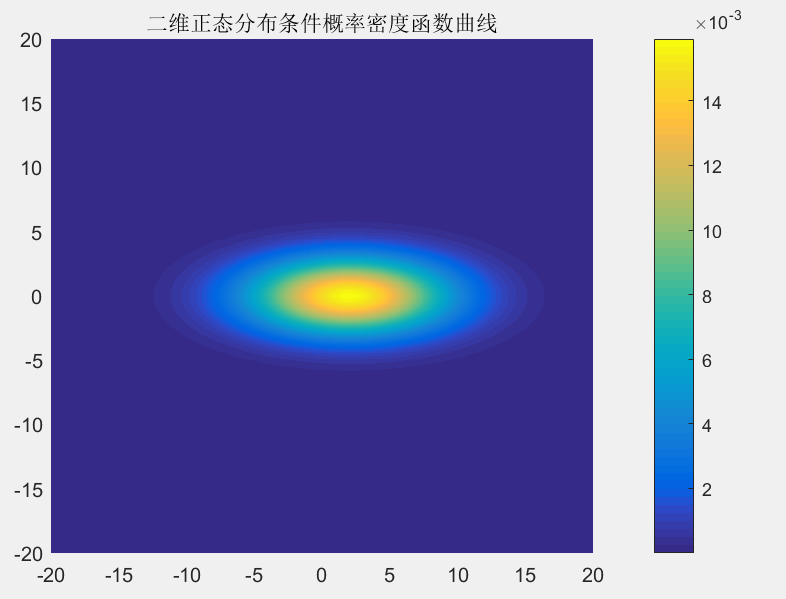图4 俯视图2
变量x的均值为2，方差为5，变量y的均值为0，方差为2，相关系数为0。 发现图像位置发生了平移，而且截面从圆形变成了椭圆。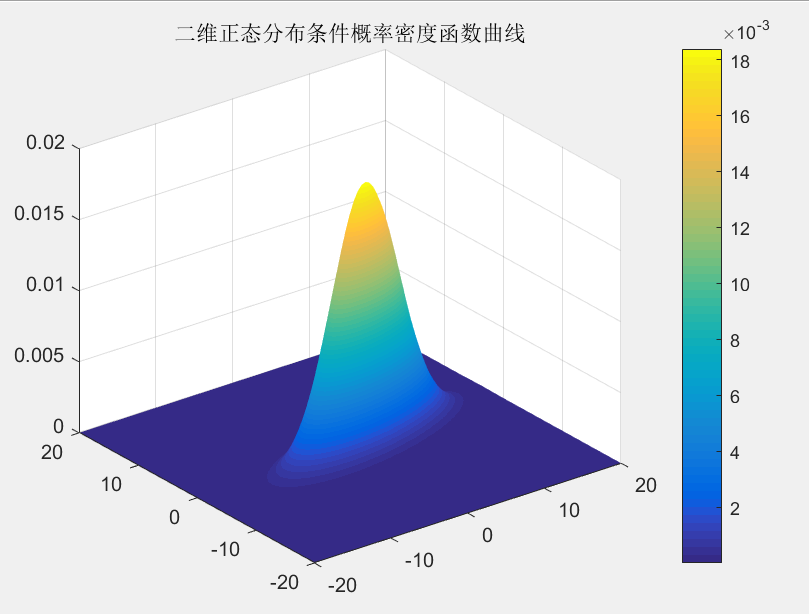图5 三维图3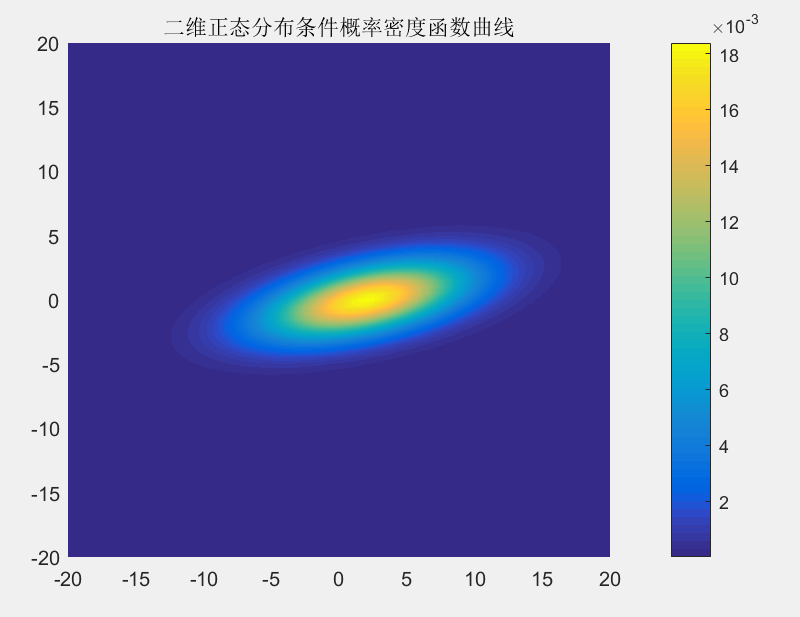图6 俯视图3
变量x的均值为2，方差为5，变量y的均值为0，方差为2，相关系数为0.5。 发现图像发生了旋转。变量x和变量y开始有同样的变化趋势。 嘿嘿。图还挺好看的。下面把代码也放上来。大家可以自行调试。
x=-20:0.5:20;
y=-20:0.5:20;
u1 = 2;          %均值
u2 = 0;
sigma1 = 5;      %方差
sigma2 = 2;
rou = 0.5;     %相关系数
mu=[-1,2];
[X,Y]=meshgrid(x,y); % 产生网格数据并处理
p = 1/(2*pi*sigma1*sigma2*sqrt(1-rou*rou)).*exp(-1/(2*(1-rou^2)).*[(X-u1).*(X-u1)/(sigma1*sigma1)-2*rou*(X-u1).*(Y-u2)/(sigma1*sigma2)+(Y-u2).*(Y-u2)/(sigma2*sigma2)]);
figure(2)
surf(X,Y,p)
colorbar
title('二维正态分布条件概率密度函数曲线');

展开全文•  当特征属性为连续值时，通常假定其值服从高斯分布（也称正态分布）。即：    而  因此只要计算出训练样本中各个类别中此特征项划分的各均值和标准差，代入上述公式即可得到需要的估计值。均值与...
1、贝叶斯介绍

我个人一直很喜欢算法一类的东西，在我看来算法是人类智慧的精华，其中蕴含着无与伦比的美感。而每次将学过的算法应用到实际中，并解决了实际问题后，那种快感更是我在其它地方体会不到的。
一直想写关于算法的博文，也曾写过零散的两篇，但也许是相比于工程性文章来说太小众，并没有引起大家的兴趣。最近面临毕业找工作，为了能给自己增加筹码，决定再次复习算法方面的知识，我决定趁这个机会，写一系列关于算法的文章。这样做，主要是为了加强自己复习的效果，我想，如果能将复习的东西用自己的理解写成文章，势必比单纯的读书做题掌握的更牢固，也更能触发自己的思考。如果能有感兴趣的朋友从中有所收获，那自然更好。
这个系列我将其命名为“算法杂货铺”，其原因就是这些文章一大特征就是“杂”，我不会专门讨论堆栈、链表、二叉树、查找、排序等任何一本数据结构教科书都会讲的基础内容，我会从一个“专题”出发，如概率算法、分类算法、NP问题、遗传算法等，然后做一个引申，可能会涉及到算法与数据结构、离散数学、概率论、统计学、运筹学、数据挖掘、形式语言与自动机等诸多方面，因此其内容结构就像一个杂货铺。当然，我会竭尽所能，尽量使内容“杂而不乱”。
1.1、摘要
贝叶斯分类是一类分类算法的总称，这类算法均以贝叶斯定理为基础，故统称为贝叶斯分类。本文作为分类算法的第一篇，将首先介绍分类问题，对分类问题进行一个正式的定义。然后，介绍贝叶斯分类算法的基础——贝叶斯定理。最后，通过实例讨论贝叶斯分类中最简单的一种：朴素贝叶斯分类。
1.2、分类问题综述
对于分类问题，其实谁都不会陌生，说我们每个人每天都在执行分类操作一点都不夸张，只是我们没有意识到罢了。例如，当你看到一个陌生人，你的脑子下意识判断TA是男是女；你可能经常会走在路上对身旁的朋友说“这个人一看就很有钱、那边有个非主流”之类的话，其实这就是一种分类操作。
从数学角度来说，分类问题可做如下定义：
已知集合：和，确定映射规则，使得任意有且仅有一个使得成立。（不考虑模糊数学里的模糊集情况）
其中C叫做类别集合，其中每一个元素是一个类别，而I叫做项集合，其中每一个元素是一个待分类项，f叫做分类器。分类算法的任务就是构造分类器f。
这里要着重强调，分类问题往往采用经验性方法构造映射规则，即一般情况下的分类问题缺少足够的信息来构造100%正确的映射规则，而是通过对经验数据的学习从而实现一定概率意义上正确的分类，因此所训练出的分类器并不是一定能将每个待分类项准确映射到其分类，分类器的质量与分类器构造方法、待分类数据的特性以及训练样本数量等诸多因素有关。
例如，医生对病人进行诊断就是一个典型的分类过程，任何一个医生都无法直接看到病人的病情，只能观察病人表现出的症状和各种化验检测数据来推断病情，这时医生就好比一个分类器，而这个医生诊断的准确率，与他当初受到的教育方式（构造方法）、病人的症状是否突出（待分类数据的特性）以及医生的经验多少（训练样本数量）都有密切关系。
1.3、贝叶斯分类的基础——贝叶斯定理
每次提到贝叶斯定理，我心中的崇敬之情都油然而生，倒不是因为这个定理多高深，而是因为它特别有用。这个定理解决了现实生活里经常遇到的问题：已知某条件概率，如何得到两个事件交换后的概率，也就是在已知P(A|B)的情况下如何求得P(B|A)。这里先解释什么是条件概率：
表示事件B已经发生的前提下，事件A发生的概率，叫做事件B发生下事件A的条件概率。其基本求解公式为：。
贝叶斯定理之所以有用，是因为我们在生活中经常遇到这种情况：我们可以很容易直接得出P(A|B)，P(B|A)则很难直接得出，但我们更关心P(B|A)，贝叶斯定理就为我们打通从P(A|B)获得P(B|A)的道路。
下面不加证明地直接给出贝叶斯定理：

1.4、朴素贝叶斯分类
1.4.1、朴素贝叶斯分类的原理与流程
朴素贝叶斯分类是一种十分简单的分类算法，叫它朴素贝叶斯分类是因为这种方法的思想真的很朴素，朴素贝叶斯的思想基础是这样的：对于给出的待分类项，求解在此项出现的条件下各个类别出现的概率，哪个最大，就认为此待分类项属于哪个类别。通俗来说，就好比这么个道理，你在街上看到一个黑人，我问你你猜这哥们哪里来的，你十有八九猜非洲。为什么呢？因为黑人中非洲人的比率最高，当然人家也可能是美洲人或亚洲人，但在没有其它可用信息下，我们会选择条件概率最大的类别，这就是朴素贝叶斯的思想基础。
朴素贝叶斯分类的正式定义如下：
1、设为一个待分类项，而每个a为x的一个特征属性。
2、有类别集合。
3、计算。
4、如果，则。
那么现在的关键就是如何计算第3步中的各个条件概率。我们可以这么做：
1、找到一个已知分类的待分类项集合，这个集合叫做训练样本集。
2、统计得到在各类别下各个特征属性的条件概率估计。即。
3、如果各个特征属性是条件独立的，则根据贝叶斯定理有如下推导：

因为分母对于所有类别为常数，因为我们只要将分子最大化皆可。又因为各特征属性是条件独立的，所以有：

根据上述分析，朴素贝叶斯分类的流程可以由下图表示（暂时不考虑验证）：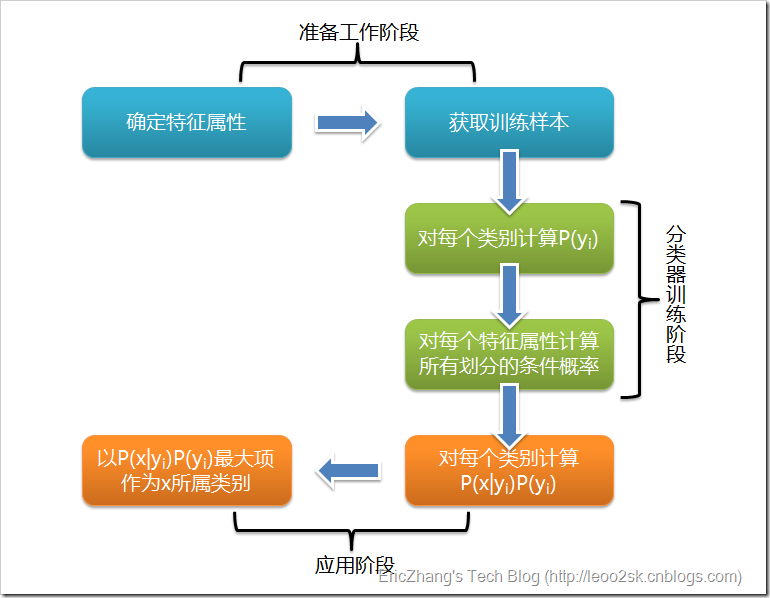可以看到，整个朴素贝叶斯分类分为三个阶段：
第一阶段——准备工作阶段，这个阶段的任务是为朴素贝叶斯分类做必要的准备，主要工作是根据具体情况确定特征属性，并对每个特征属性进行适当划分，然后由人工对一部分待分类项进行分类，形成训练样本集合。这一阶段的输入是所有待分类数据，输出是特征属性和训练样本。这一阶段是整个朴素贝叶斯分类中唯一需要人工完成的阶段，其质量对整个过程将有重要影响，分类器的质量很大程度上由特征属性、特征属性划分及训练样本质量决定。
第二阶段——分类器训练阶段，这个阶段的任务就是生成分类器，主要工作是计算每个类别在训练样本中的出现频率及每个特征属性划分对每个类别的条件概率估计，并将结果记录。其输入是特征属性和训练样本，输出是分类器。这一阶段是机械性阶段，根据前面讨论的公式可以由程序自动计算完成。
第三阶段——应用阶段。这个阶段的任务是使用分类器对待分类项进行分类，其输入是分类器和待分类项，输出是待分类项与类别的映射关系。这一阶段也是机械性阶段，由程序完成。
1.4.2、估计类别下特征属性划分的条件概率及Laplace校准
这一节讨论P(a|y)的估计。
由上文看出，计算各个划分的条件概率P(a|y)是朴素贝叶斯分类的关键性步骤，当特征属性为离散值时，只要很方便的统计训练样本中各个划分在每个类别中出现的频率即可用来估计P(a|y)，下面重点讨论特征属性是连续值的情况。
当特征属性为连续值时，通常假定其值服从高斯分布（也称正态分布）。即：

而
因此只要计算出训练样本中各个类别中此特征项划分的各均值和标准差，代入上述公式即可得到需要的估计值。均值与标准差的计算在此不再赘述。
另一个需要讨论的问题就是当P(a|y)=0怎么办，当某个类别下某个特征项划分没有出现时，就是产生这种现象，这会令分类器质量大大降低。为了解决这个问题，我们引入Laplace校准，它的思想非常简单，就是对没类别下所有划分的计数加1，这样如果训练样本集数量充分大时，并不会对结果产生影响，并且解决了上述频率为0的尴尬局面。
1.4.3、朴素贝叶斯分类实例：检测SNS社区中不真实账号
下面讨论一个使用朴素贝叶斯分类解决实际问题的例子，为了简单起见，对例子中的数据做了适当的简化。
这个问题是这样的，对于SNS社区来说，不真实账号（使用虚假身份或用户的小号）是一个普遍存在的问题，作为SNS社区的运营商，希望可以检测出这些不真实账号，从而在一些运营分析报告中避免这些账号的干扰，亦可以加强对SNS社区的了解与监管。
如果通过纯人工检测，需要耗费大量的人力，效率也十分低下，如能引入自动检测机制，必将大大提升工作效率。这个问题说白了，就是要将社区中所有账号在真实账号和不真实账号两个类别上进行分类，下面我们一步一步实现这个过程。
首先设C=0表示真实账号，C=1表示不真实账号。
1、确定特征属性及划分
这一步要找出可以帮助我们区分真实账号与不真实账号的特征属性，在实际应用中，特征属性的数量是很多的，划分也会比较细致，但这里为了简单起见，我们用少量的特征属性以及较粗的划分，并对数据做了修改。
我们选择三个特征属性：a1：日志数量/注册天数，a2：好友数量/注册天数，a3：是否使用真实头像。在SNS社区中这三项都是可以直接从数据库里得到或计算出来的。
下面给出划分：a1：{a<=0.05, 0.05<a<0.2, a>=0.2}，a1：{a<=0.1, 0.1<a<0.8, a>=0.8}，a3：{a=0（不是）,a=1（是）}。
2、获取训练样本
这里使用运维人员曾经人工检测过的1万个账号作为训练样本。
3、计算训练样本中每个类别的频率
用训练样本中真实账号和不真实账号数量分别除以一万，得到：

4、计算每个类别条件下各个特征属性划分的频率

5、使用分类器进行鉴别
下面我们使用上面训练得到的分类器鉴别一个账号，这个账号使用非真实头像，日志数量与注册天数的比率为0.1，好友数与注册天数的比率为0.2。

可以看到，虽然这个用户没有使用真实头像，但是通过分类器的鉴别，更倾向于将此账号归入真实账号类别。这个例子也展示了当特征属性充分多时，朴素贝叶斯分类对个别属性的抗干扰性。
1.5、分类器的评价
虽然后续还会提到其它分类算法，不过这里我想先提一下如何评价分类器的质量。
首先要定义，分类器的正确率指分类器正确分类的项目占所有被分类项目的比率。
通常使用回归测试来评估分类器的准确率，最简单的方法是用构造完成的分类器对训练数据进行分类，然后根据结果给出正确率评估。但这不是一个好方法，因为使用训练数据作为检测数据有可能因为过分拟合而导致结果过于乐观，所以一种更好的方法是在构造初期将训练数据一分为二，用一部分构造分类器，然后用另一部分检测分类器的准确率。

2、MATLAB的程序代码：

%%%%%%%%%%%%%%%%%%%%%%%%%%%%%%%%%%%%%%%%%%%%%%%%%%%
%By Shelley from NCUT，April 14th 2011
%Email:just_for_h264@163.com
%此程序利用贝叶斯分类算法，首先对两类样本进行训练，
%进而可在屏幕上任意取点，程序可输出属于第一类，还是第二类
%%%%%%%%%%%%%%%%%%%%%%%%%%%%%%%%%%%%%%%%%%%%%%%%%%%
clear;
close all

%读入两类训练样本数据
%求两类训练样本的均值和方差
u1=mean(Sample1);
u2=mean(Sample2);
sigm1=cov(Sample1);
sigm2=cov(Sample2);
%计算两个样本的密度函数并显示
x=-20:0.5:40;
y= -20:0.5:20;
[X,Y] = meshgrid(x,y);
F1 = mvnpdf([X(:),Y(:)],u1,sigm1);
F2 = mvnpdf([X(:),Y(:)],u2,sigm2);
P1=reshape(F1,size(X));
P2=reshape(F2,size(X));
figure(2)
surf(X,Y,P1)
hold on
surf(X,Y,P2)
colorbar
title('条件概率密度函数曲线');
%%%%%%%%%%%%%%%%%%%%%%%%%%%%%%%%%%%%%%%%%%%%%%%%%%
%以下为测试部分
%利用ginput随机选取屏幕上的点（可连续取10个点）
%程序可根据点的位置自动地显示出属于那个类
%%%%%%%%%%%%%%%%%%%%%%%%%%%%%%%%%%%%%%%%%%%%%%%%%%%
pw1=0.4;pw2=0.6;

figure(1)
plot(Sample1(:,1),Sample1(:,2),'r.')
hold on
plot(Sample2(:,1),Sample2(:,2),'b.')

for i=1:10
[u,v]=ginput(1);
plot(u,v,'m*');
P1=pw1*mvnpdf([u,v],u1,sigm1);
P2=pw2*mvnpdf([u,v],u2,sigm2);
hold all
if(P1>P2)
disp('it belong to the first class');
else
disp('it belong to the second class');
end;
end
%%%%%%%%%%%%%%%%%%%%%%%%%%%%%%%%%%%%%%%%%%%%%%%%%%%其结果如下：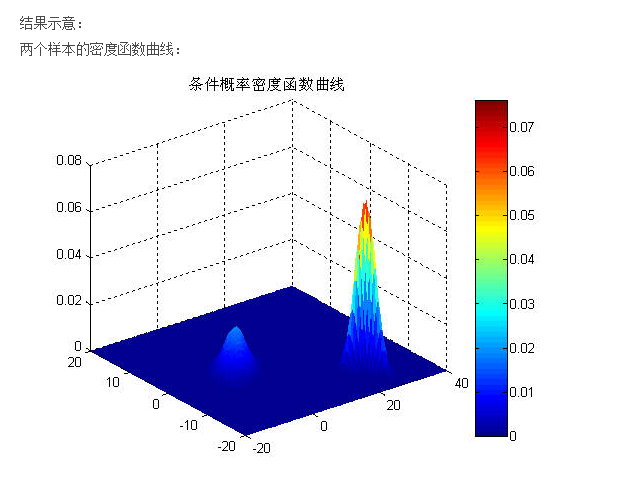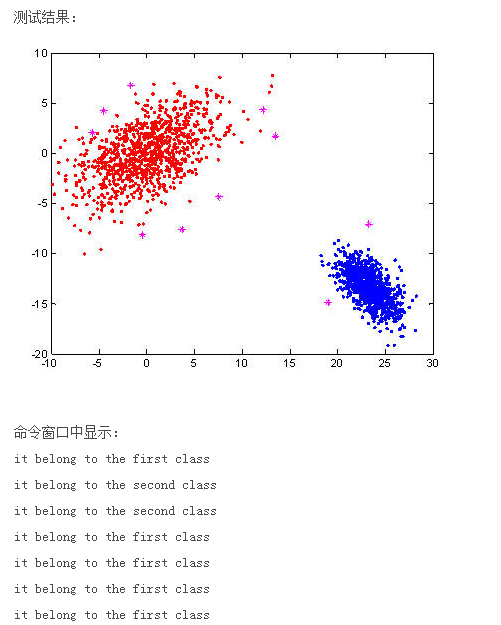3、MATLAB进行二维正太分布图
为什么要介绍这个原因，是因为最小风险贝叶斯里面的那个P(X|Wi)是二维正态分布概率密度，其x轴是每个样本的属性，y轴是每个样本中属性对于的个数。其是程正态分布的，其中的期望值是所有属性对应个数加起来的平均值。则其二维正态分布概率密度公式是：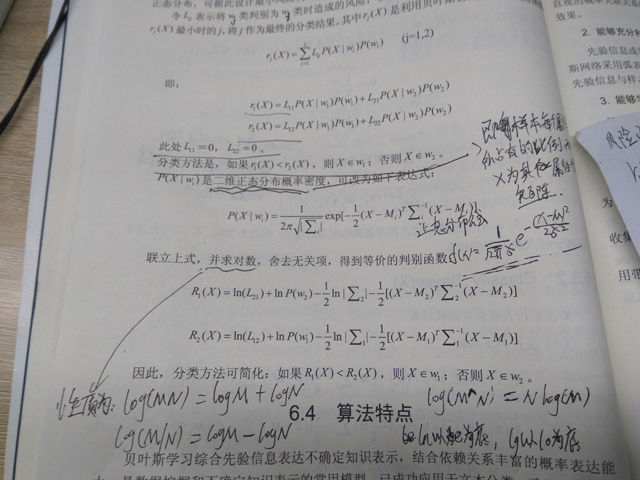clc
clear
close all

mu=[0,0];% 均值向量
Sigma=[1 0.8;0.8 1];% 协方差矩阵
[X,Y]=meshgrid(-3:0.1:3,-3:0.1:3);%在XOY面上，产生网格数据
p=mvnpdf([X(:) Y(:)],mu,Sigma);%求取联合概率密度，相当于Z轴
p=reshape(p,size(X));%将Z值对应到相应的坐标上

figure
set(gcf,'Position',get(gcf,'Position').*[1 1 1.3 1])

subplot(2,3,[1 2 4 5])
surf(X,Y,p),axis tight,title('二维正态分布图')
subplot(2,3,3)
surf(X,Y,p),view(2),axis tight,title('在XOY面上的投影'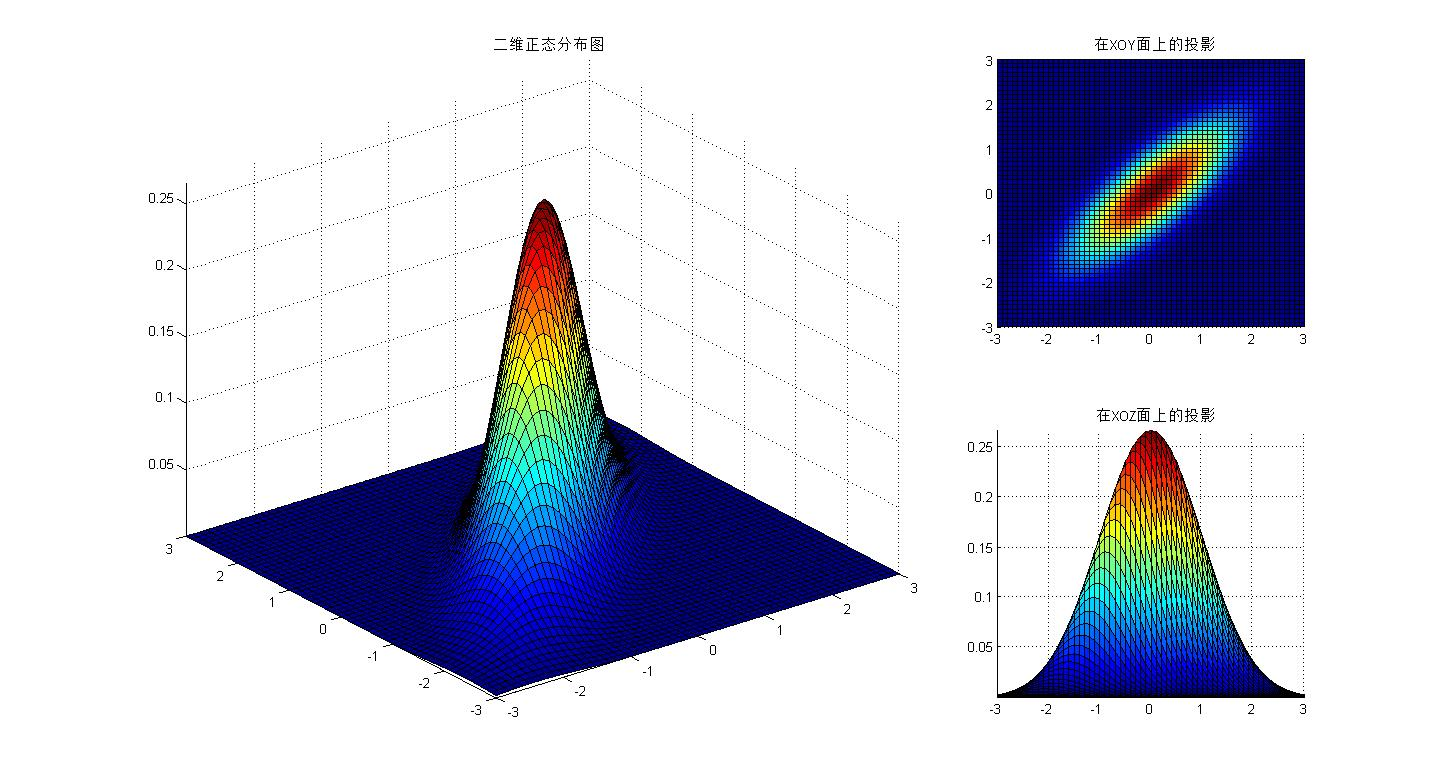3、最小风险贝叶斯分类器的构建
其代码是：

%%%%%%%%%%%%%%%%%%%%%%%%%%%%%%%%%%%%%%%%%%%%%
%功能：演示贝叶斯学习算法在计算机视觉中的应用
%基于贝叶斯学习实现目标分类；
%其是真实训练跟测试数据都是k_d-k_s=120-11=109
%环境：Win7，Matlab2012b
%Modi: NUDT-VAP
%时间：2014-02-04
%%%%%%%%%%%%%%%%%%%%%%%%%%%%%%%%%%%%%%%%%%%%%
close all
clear all
%加载背景和行人样本
%随机划分训练样本和测试样本
for j=1:10
k_s=11;
k_d=120;
hm_tr(250,252)=0;    %这只初始化了一个元素
hm_test(250,252)=0;
% ceshi=ones(size(hm_test));
[hm_tr,hm_test]=randQ(human);
bg_tr(250,252)=0;
bg_test(250,252)=0;
[bg_tr,bg_test]=randQ(background);   %这里的测试数据值跟训练的数据值是不一样的，所以这里要使用区域范围来把测试数据归为训练数据
%设计最小风险贝叶斯分类器
M_hm=hm_tr(1,k_s:k_d);
for i=2:250
M_hm=M_hm+(hm_tr(i,k_s:k_d));  %每一列的数据进行累加
end
M_hm=M_hm/250;                 %累加求平均值
M_bg=bg_tr(1,k_s:k_d);
for i=2:250
M_bg=M_bg+(bg_tr(i,k_s:k_d));
end
M_bg=M_bg/250;
E_hm(k_d-k_s+1,k_d-k_s+1)=0;
E_bg(k_d-k_s+1,k_d-k_s+1)=0;
for i=1:250
E_hm=E_hm+(hm_tr(i,k_s:k_d)-M_hm)'*(hm_tr(i,k_s:k_d)-M_hm);
E_bg=E_bg+(bg_tr(i,k_s:k_d)-M_bg)'*(bg_tr(i,k_s:k_d)-M_bg);
end
E_hm=E_hm/250;  %这里不是归一化
E_bg=E_bg/250;

%分类测试
%设计判决函数并分类
flag_hm(250)=0;
flag_bg(250)=0;
for i=1:250
dk_hm=log(0.5)+log(0.95)-log(abs(det(E_bg)))/2-((hm_test(i,k_s:k_d)-M_bg)*inv(E_bg)*(hm_test(i,k_s:k_d)-M_bg)')/2;   %其中的0.5是背景判为行人的风险；1.5为行人判为背景的风险；出现背景的先验概率为0.95；出现行人的先验概率为0.05；
dk_bg=log(1.5)+log(0.05)-log(abs(det(E_hm)))/2-((hm_test(i,k_s:k_d)-M_hm)*inv(E_hm)*(hm_test(i,k_s:k_d)-M_hm)')/2;
if(dk_hm<=dk_bg)
flag_hm(i)=1;
else flag_hm(i)=0;
end
end
s2_hm=sum(flag_hm);
for i=1:250
%因为只有方阵才有逆矩阵
h=inv(E_bg);
dk_hm=log(0.5)+log(0.95)-log(abs(det(E_bg)))/2-((bg_test(i,k_s:k_d)-M_bg)*inv(E_bg)*(bg_test(i,k_s:k_d)-M_bg)')/2;
dk_bg=log(1.5)+log(0.05)-log(abs(det(E_hm)))/2-((bg_test(i,k_s:k_d)-M_hm)*inv(E_hm)*(bg_test(i,k_s:k_d)-M_hm)')/2;
if(dk_hm>dk_bg)
flag_bg(i)=1;
else flag_bg(i)=0;
end
end
%统计分类结果
s2_bg=sum(flag_bg);
err_hg(j)=(250-s2_hm)/250;
err_gh(j)=(250-s2_bg)/250;
end
ERR_HG=0;
ERR_GH=0;
for j=1:10
ERR_HG=ERR_HG+err_hg(j);
ERR_GH=ERR_GH+err_gh(j);
end
ERR_HG=ERR_HG/10
ERR_GH=ERR_GH/10



1、贝叶斯分类器的matlab实现
2、算法杂货铺——分类算法之朴素贝叶斯分类(Naive Bayesian classification)
3、MATLAB二维正态分布图
4、数据是正态分布的例子，有图

展开全文• % 二联合概率分布 %方法一：调包 mu = zeros(1,2); % 均值 sigma = [1 , 0.9; 0.9 , 1]; % 协方差 rng('default') % For reproducibility X = mvnrnd(mu,sigma,1000); y = mvnpdf(X,mu,sigma); figure scatter...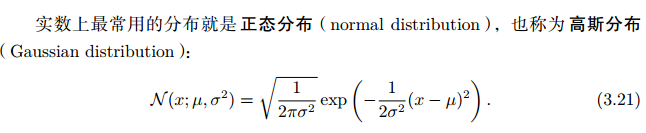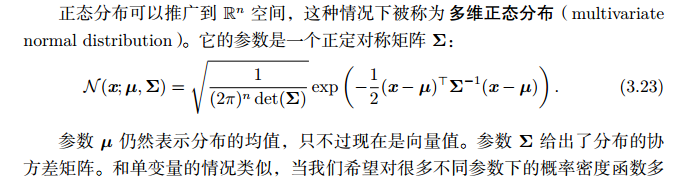clc;
close all; clear;
% 多维联合高斯概率分布
%%
% 二维联合概率分布

%方法一：调包
mu = zeros(1,2);     % 均值
sigma = [1  , 0.9;
0.9  , 1];  % 协方差
rng('default')  % For reproducibility
X = mvnrnd(mu,sigma,1000);

y = mvnpdf(X,mu,sigma);
figure
scatter3(X(:,1),X(:,2),y, '.')
xlabel('X1')
ylabel('X2')
zlabel('Probability Density')
%%
%方法二：直接上公式
mu2 = mean(X);
sigma2 = cov(X);
sigma3 = (X-mu2)'*(X-mu2) / length(X);  % 用公式计算协方差 E[(X-mu)'(X-mu)]

y2 = sqrt(1/((2*pi)^length(mu2)*det(sigma2))) * exp(-1/2*(X-mu2)*inv(sigma2)*(X-mu2)');
y3 = zeros(1000, 1);
for i = 1: 1000
y3(i) =  y2(i, i);   % 这里是重点！
end
figure
scatter3(X(:,1),X(:,2),y3, '.')
xlabel('X1')
ylabel('X2')
zlabel('Probability Density')


MATLAB不配拥有代码段格式吗。。。。。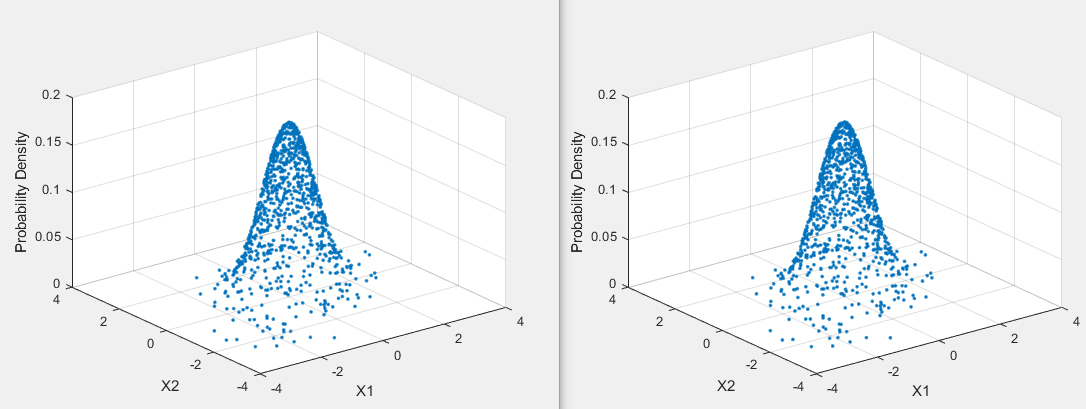展开全文• 本篇文章主要讲解在matlab环境下，如何随机生成满足高斯分布的样本点。  众所周知，高斯分布是一种很常见的数据分布，也是很多实际例子中出现最多的一种分布。我们今天就来学习在matlab环境下绘制出随机分布的满足...
       本篇文章主要讲解在matlab环境下，如何随机生成满足高斯分布的样本点。
众所周知，高斯分布是一种很常见的数据分布，也是很多实际例子中出现最多的一种分布。我们今天就来学习在matlab环境下绘制出随机分布的满足高斯分布的数据点。
1.二维空间二分类问题

%%参数设置
mean = [2 3];   %%均值
SIGMA = [1 0;0 2];  %%协方差矩阵,假设各维度独立，故可设为对角阵
r = mvnrnd(mean,SIGMA,100);  %%最后一个参数表示生成该类100个样本点
plot(r(:,1),r(:,2),'r+');
grid on;               %%描绘出网格
hold on;               %%停留在图中，
mean = [4 2];
SIGMA = [1 0;0 2];
r2 = mvnrnd(mean,SIGMA,100);
plot(r2(:,1),r2(:,2),'b*')
grid on;
运行结果：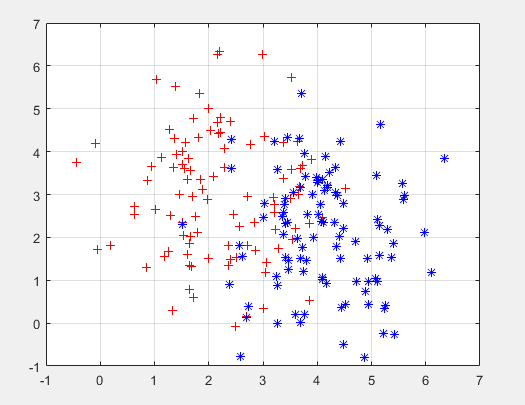以上很多都是绘图的一些基本命令，最重要的是mvnrnd(mean,SIGMA,100)这个函数，第一个参数表示随机生成的样本点的均值，第二个参数表示这些样本点的协方差矩阵，我们可以假设各维度相互独立，也就是设为对角阵（只有主对角线上的元素不为0），第三个参数100表示生成100个样本点。plot就是绘制图像的函数，前两个参数分别对应的是样本点的第一维和第二维的值，而第三个参数表示'r+',用红色的+标记。那么我们可用的颜色和标记符号有哪些呢？ 请参照下面这张图就好了。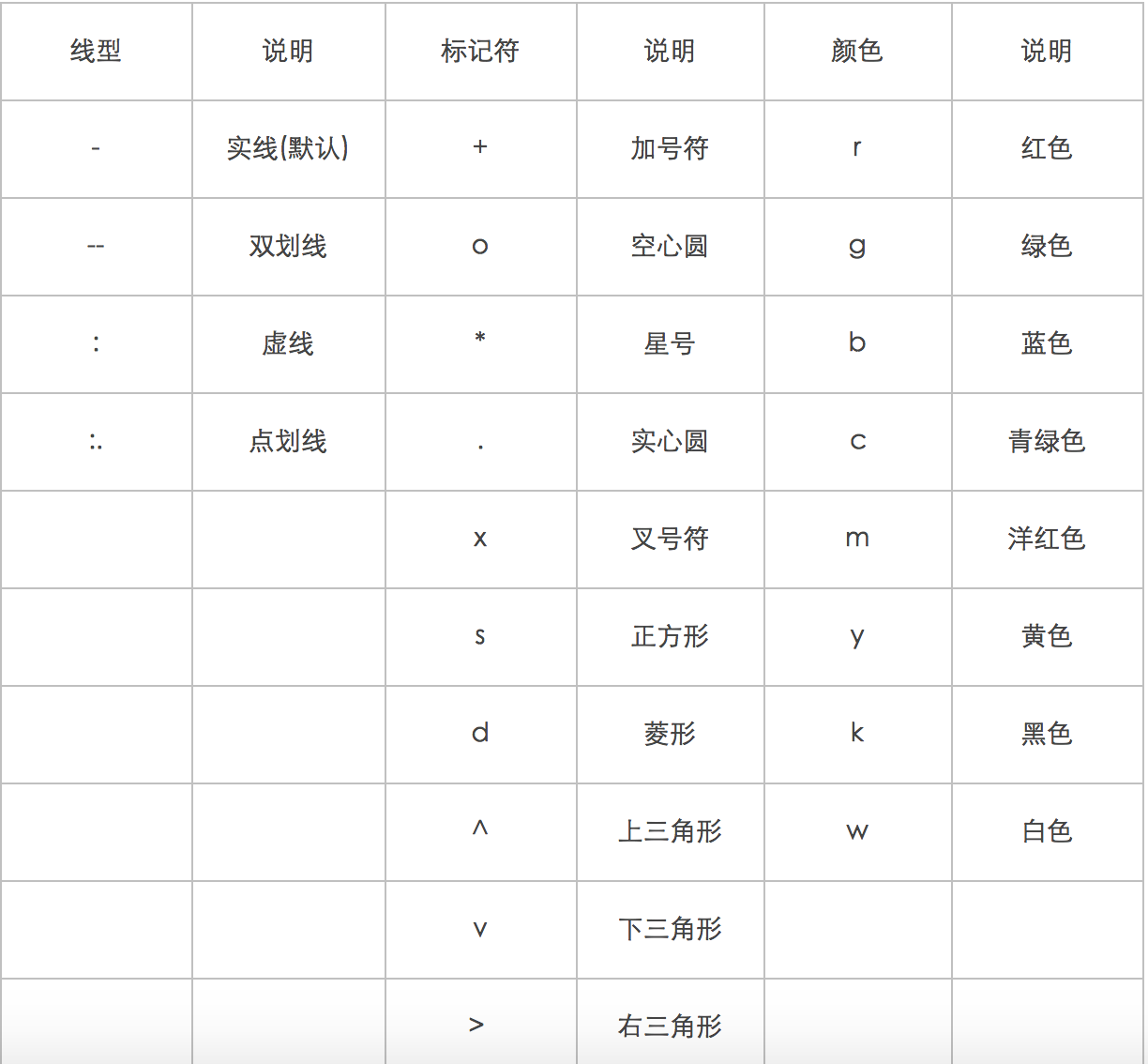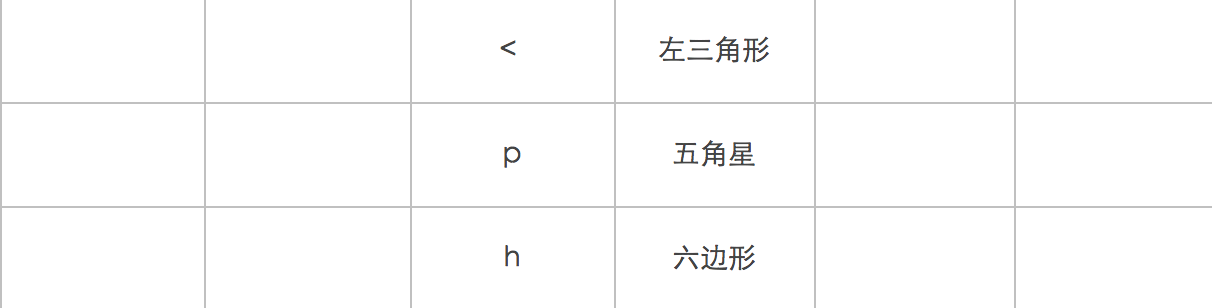2.二维空间三分类问题

mean = [2 3];   %%均值
SIGMA = [1 0;0 2];  %%协方差矩阵,假设各维度独立，故可设为对角阵
r = mvnrnd(mean,SIGMA,100);  %%最后一个参数表示生成该类10个样本点
plot(r(:,1),r(:,2),'r+');
grid on;               %%描绘出网格
hold on;               %%停留在图中，
mean = [7 8];
SIGMA = [1 0;0 2];
r2 = mvnrnd(mean,SIGMA,100);
plot(r2(:,1),r2(:,2),'b*')
grid on;
hold on;
mean = [4 6]
SIGMA = [1 0;0 4];
r3 = mvnrnd(mean,SIGMA,100);
plot(r3(:,1),r3(:,2),'gs')
运行结果：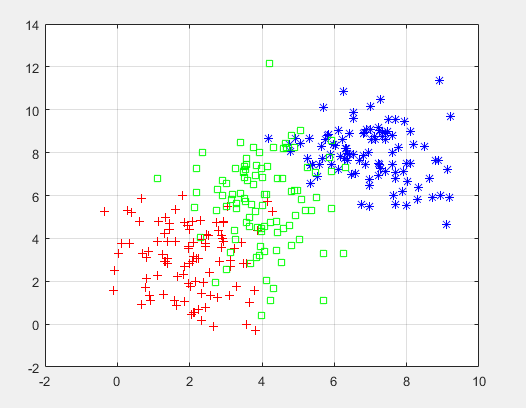3.三维三分类问题

mean = [2 3 4];   %%均值
SIGMA = [1 0 0;0 2 0;0 0 3]  %%协方差矩阵,假设各维度独立，故可设为对角阵
r = mvnrnd(mean,SIGMA,10);  %%最后一个参数表示生成该类10个样本点
plot3(r(:,1),r(:,2),r(:,3),'r+');
grid on;               %%描绘出网格
hold on;               %%停留在图中，
mean = [7 8 9];
SIGMA = [1 0 0;0 2 0;0 0 4];
r2 = mvnrnd(mean,SIGMA,10);
plot3(r2(:,1),r2(:,2),r2(:,3),'b*')
grid on;
hold on;
mean = [4 8 13]
SIGMA = [1 0 0;0 2 0;0 0 4];
r3 = mvnrnd(mean,SIGMA,10);
plot3(r3(:,1),r3(:,2),r3(:,3),'gs')
结果：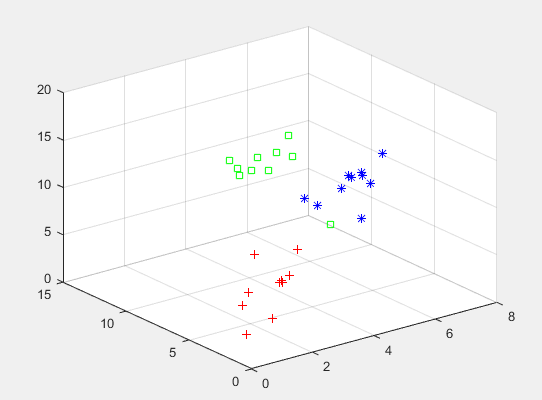这里需要注意的是，绘制三维图像时，与二维平面图唯一的区别是plot函数，变成了plot3函数，参数的个数也增加了一维度。
如有问题，欢迎私信和QQ交流~
展开全文• 利用 MATLAB 绘制正态分布曲线正文 欢迎学习交流！ 个人网站：https://zephyrhours.github.io/ 邮箱：zengf.hou@bit.edu.cn 声明： 下面代码基于Matlab 2018b，如果您的论文中使用了下面代码，请添加引用： ...windows
• 维正态随机变量概率密度函数三维图的matlab实现 1.二维正态随机变量 二维正态随机变量是最常见的一种二维随机变量分布。其联合概率密度函数为： p(x,y)=12πσXσY1−r2⋅exp{−12(1−r2)[(x−mX2)σX2−2r(x−mX)...
• 本文首先给出正态分布概率密度函数（The normal distribution probability density function）的公式和标准正态分布概率密度函数的公式，然后通过normpdf( )生成标准正态分布概率密度函数的数据，然后通过plot( )...
• 首先，把二维正态分布密度函数的公式贴这里 这只图好大啊~~ 但是上面的那个是多维正态分布的密度函数的通式，那个n阶是对称正定方阵叫做协方差矩阵，其中的x,pi,u都是向量形式。虽然这个式子很酷，但是用...
• Matlab实现高斯函数的三维显示1.前言2.代码3.结果展示 1.前言 最近学习中用到了高斯函数（二维高斯分布），特记录下实现代码及一些注意事项。 2.代码 %设置渐变色：figure => 编辑 => 图形属性 %去掉网格,并使...
• 本篇博文记录使用MATLAB绘制二维高斯函数的三维图。 用到的MATLAB函数——mesh()（绘制三维线框图）和surf()（绘制三维表面图）。 MATLAB命令窗口输入&gt;&gt; doc 函数名，可以查看相关函数的帮助文档。...高斯函数
• 首先我们有二维正态分布： X,Y∼BVN(μx,μy,σx2,σy2,ρxy)X,Y\sim \mathbf{BVN}(\mu_x,\mu_y,\sigma_x^2,\sigma_y^2,\rho_{xy})X,Y∼BVN(μx​,μy​,σx2​,σy2​,ρxy​) 取对数之后我们会得到二维对数正态...协方差 数学
• 这里是一个简单的实现代码 x=linspace（-5,5,50); %生成负五到五之间的五十个数，行矢量 y=normpdf(x,0,1); plot(x,y,‘k’);...第一，正态分布概率密度函数的公式如下图。其中，μ为平均值（mean...
• 绘制二元正态分布的密度函数图和等高线图，mesh函数（密度图）和contour函数（等高线）
• ** 正态分布 准则：设 ，（1）画出其密度函数曲线 ；（2）分别对 ， ， 进行填充；（3）分别求出随机变量 落在这个区间内的概率；（4）产生 个随机数，计算其分别落在这个区间的频率.** 2. 问题理论分析： 个...统计学
• 在这个例子中，函数 ellipsedata 构造了个 100 个点的椭圆，每个椭圆代表对应于 1、2 和 3 标准差的轮廓曲线，高斯分布的协方差矩阵由 [4,1;1,1] 给定，平均值给定通过 [3,3]。 elpt=ellipsedata([4,1;1,1],[3,3]...
• 一、 三维绘图 p = 0: pi/10: 20*pi; x = cos(p); y = sin(p); z = p; plot3(x,y,z) x = -2:.2:2; %有-2为起点，2为递增步长，2为终止点 y = -1:.2:1; [xx, yy] = meshgrid(x, y); %用于从数组x和y产生网格,...
• ## 正态分布3σ原则

千次阅读 2021-06-23 09:14:26
正态分布是具有两个参数μ（数学期望）和σ2（标准差）的连续型随机变量的分布，第一参数μ是遵从正态分布的随机变量的均值，第二个参数σ2是此随机变量的方差，所以正态分布记作N(μ，σ2 )。遵从正态分布的随机...
• 冻结屏幕高宽比，使得一个三维对象的旋转不会改变坐标轴的刻度显示 13. axis off 关闭所有的坐标轴标签、刻度、背景 14. axis on 打开所有的坐标轴标签、刻度、背景 希腊字母等特殊...
• 如何产生正态分布的随机数？ 添加评论  分享 按投票排序按时间排序 28 个回答 86赞同 反对，不会显示你的姓名 Milo Yip，游戏程序员、《游戏引擎架构》...
• 因为Pythonista没有scipy科学计算包，遇上需求标准正态累积分布函数的时候就只能抓瞎，为此决定自己写一个。累积分布函数（Cumulative Distribution Function，CDF）就是概率密度函数（Probability De...
• matlab生成二服从高斯分布的数据 ...由于实验需要，需要生成两类模式的数据，同时这两类数据要服从正态分布（高斯分布）。  使用matlab来实现： mu = [2 3]; SIGMA = [1 0; 0 2]; r = mvnrnd(mu,SI算法 C
• n= 5 ; m = 0 ; s ...第个参数代表的是按照列求标准差还是按照行求标准差， std (A, 1 , 1 )代表的是按照列求标准差， std (A, 1 , 2 )代表的是按照行求标准差。 结果： std函数参考 ...
• clear;close all;clc; hold on; grid; for n=1:100 fn=(1+1/n)^n; plot(n,fn,'r.','markersize',10); pause(0.1); end clear;close all;clc; hold on; axis([0,100,0,2]) grid; ... pause(0.
• 基于Python的二高斯3D显示 区别于MATLAB 使用Python绘图Python
• ## 多元正态分布的极大似然估计

万次阅读 多人点赞 2018-06-20 13:53:12
多元正态分布的极大似然估计 1. 一元正态分布的密度函数 一元正态分布的密度函数表示为： f(x)=1(2π)−−−−√σe−(x−μ)22σ2f(x)=1(2π)σe−(x−μ)22σ2f(x) = \frac{1}{\sqrt {(2 \pi)} \sigma} e^{......

# matlab三维正态分布matlab 订阅